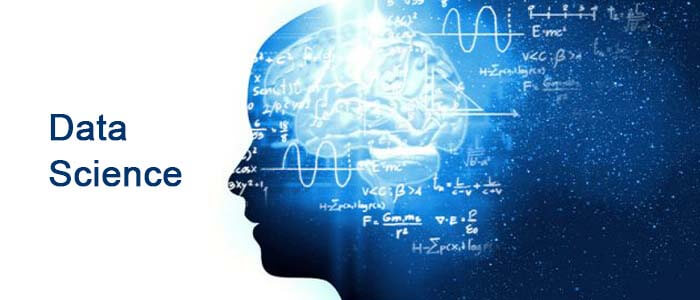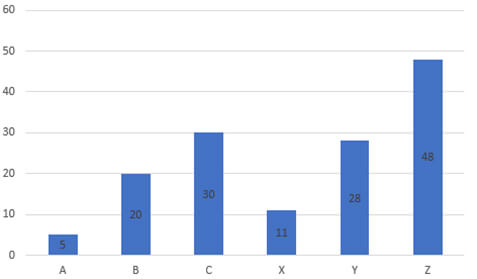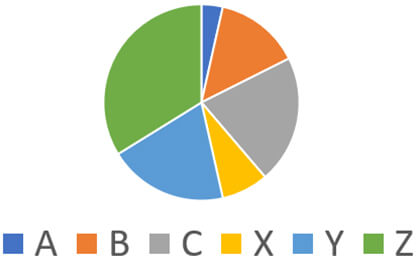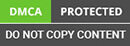﻿ Complete Guide to start Career in Data Science.

Looking for an Expert Development Team? Take two weeks Trial! Try Now

•Fill the details and We’ll get back to you soon# How to Start in Data Science?A frequent question asked by beginners in any field is how to start? In this post, the definition of the data science field will be discussed in addition to giving a guide about how to start in this field.

Data science can be defined as the field of analyzing data for making future decisions. Within this definition, there are 2 keywords and 1 keyphrase:

• Analyze
• Data
• Future Decisions

Data science will be explained based on the 3 items listed above. We can start with data.

### Data

The word data is available in the name of the field data science and this proves how important is the data for such a field. Generally, data is one of the most important factors for solving data science problems. If the data is available, then we can go ahead. Otherwise, we have to stop and think about how to get the data. This is the first experience you should have in the field of data science which is data collection. I prefer to start using the available data and not dive into the data collection stage directly.

Data is available in different types. This includes numbers, text, voice, image, video, and so on. Unfortunately, there is no single way to work with all of these types. For example, the techniques that work with the text data are different from those applied for the image data. As a result, before you start working in data science you need to focus on a specific type of data, at least at the beginning. Later, you can learn how to work on different types of data.

Summarizing the previous 2 paragraphs, you first need to define what data type you will work on when starting in data science and then learn how to collect data. Note that data collection can be simple as just downloading a dataset of the type you are using. That is you use an existing dataset. Also, data collection might be complex as preparing your dataset from scratch.

For example, some people work on analyzing the tweets posted on Twitter about a given topic. This topic might be assessing the people reviews about product X and see how people are satisfied. You might be working on a topic that no one else worked on and thus you have to collect the data about the product X yourself. Thus, you have to access the Twitter API and collect all tweets mentioning the product X. After downloading the tweets, there are some challenges to overcome such as unifying the format of all data samples you collect and removing the samples that might not be irrelevant or sometimes called noise. There are noise removal techniques that help to get rid of such samples.

After the data is ready, then we can start analyzing it as discussed in the next section.

### Analyze Data

When the data is collected, then it is available in a raw format. We are not interested in just the raw data but in the information inside it. Yes. Data has information but we need to do some efforts to reach such information. Data analysis is about summarizing the raw data to get pieces of information. How to summarize the data? This is by calculating some statistics and this is the next experience to acquire. You should know the statistics. Let's have an example.

Assume there is an online store from which people are buying 6 products A, B, C, X, Y, and Z. The raw data might be the number of units sold per each product in a single day. By accessing the users' transactions in the database of the store, here is the raw data consisting of 142 characters where each character means its corresponding product is sold only once. Think of how to find useful information from that raw data!

CYZZAZYCAZBCYZABCZYAZZBYCZAYBCZYBCZYBZYCZYBCZYCZBYCZBCZZZCYBZCCZZCY BCZYZBZYCYBZCYZZZYCZYBCZZYXBZCXBXZCZYXZBYCXZZBYCXZYXCZZXBXYCZZXZCYZBXZYCZZC

One simple way is to start counting the number of units sold per each product. From the raw data, the sold counts are as given below. Thus, rather than working with a string of length 142, we are just working with 6 numbers.

5 A 20 B 30 C 11 X 28 Y 48 Z

Based on the counts, there are some pieces of information in our hands. For example, product A has the lowest sale, product Z has the highest sale, and products C and Y have nearly equal sales.

Note that calculating the statistics is not enough and you have to present these statistics in visualized forms, if possible because it is a friendly way of capturing information easily. A bar graph is created in the next figure to show the number of sold units of the 6 products. From the graph, you can easily know that the number of sold units from product A is very small compared to the product Z.The bar graph is useful for comparing the number of each 2 products together. This does not tell how the product is doing compared to the total number of units sold. For that purpose, we can use the pie chart as illustrated in the next figure. From this figure, you can easily deduce that the number of units sold from product Z is about 30% of the total number of units sold by the store while product A is just about 3%. There are many other statistics to calculate and visualize.You can also notice that the units from product X are sold at the end of the day and the ones from product ‘A’ are sold at the beginning of the day. This can also be useful information to understand the situation.

Well. We analyzed the data using some statistics and visualized them. How these statistics are calculated and visualized? Many tools help the data scientist and you need to select the tool that fits your needs. For example, I used Microsoft Excel for working with the previous data and creating the visualizations. For another type of problem, the data might not be suitable for Excel and thus we need to use another tool.

Generally, programming is the preferred way to work in data science. The reason is that you can do whatever you need by writing code compared to using just the built-in functions available in tools such as Excel. I do not know a data scientist that does not know how to program. You can freely select your best language of Java web development but the one I prefer is Python because it has many simple-to-use libraries that help you fulfill whatever you want to do easily. Still, you can use other languages like C or Java if you prefer. Others might prefer R or MATLAB. No problem to have experience in more than one tool. It does not hurt.

Remember that analyzing the data is about calculating statistics to summarize the data. Because there are different types of data, then there are different kinds of calculating the statistics for such data. Talking about the images, for example, there are some special techniques to summarize them such as histogram of oriented gradients, local binary patterns, and others. You can apply these techniques using the tool you use (e.g. Python).

When working on a given data, you are who decide which algorithm to use. That is you might use local binary patterns for summarizing your data. This selection might or might not be right. If not right, you have to study the data again to decide the best technique for use. This takes time. Deep learning, which is an extension to machine learning, automates the process of summarizing the raw data.

These techniques perform mathematical operations on the data until summarizing it. As a result, you need a good knowledge of mathematics. These techniques accept an image of thousands of pixels and just return a vector that might include only tens of hundreds of elements.

Sometimes the returned vector by these techniques will need some processing to increase its quality. The techniques are responsible for just keeping the good elements and removing the bad ones. To be able to manipulate the returned vector, knowledge of linear algebra is preferred.

Up to this time, the data is summarized using the data analysis techniques. If you would like to start your career in data science, I prefer just focusing on a data type and study its techniques and then expand your knowledge.

After preparing the data and analyzing it, we can talk about the prediction stage in the next section.

### Future Prediction

Data science is about summarizing the raw data in a form that makes it easy for us to make predictions. Regarding the example discussed, the prediction stage can help us know which of the 6 products could be sold if a new customer entered the store.

A very simple way to make predictions is to first calculate the probability of the sold units across all products. This is by dividing the number of sold units in each product by the total number of sold units which is 142 as given below. Based on these calculations, we know that the next product to be sold may be Z with a percentage of 33.8%, C with 21.1%, Y with 19.7%, and so on.

A: 5/142 = 0.035 20 B = 20/142 = 0.1408 30 C = 30/142 = 0.211 11 X = 11/142 = 0.077 28 Y = 28/142 = 0.197 48 Z = 48/142 = 0.338

When the data is complex, the prediction stage is not just about calculating the probability by dividing the number of past occurrences for a given product over the total number of occurrences. There is a field called machine learning that is responsible for building algorithms that can deduce the complex relationships in the data to accurately predict the output. These algorithms include artificial neural networks, random forest, support vector machine, and more.

These algorithms can be viewed as a mathematical function that accepts inputs (which are the statistics calculated while analyzing the data) and the outputs which are the expected outcomes for the inputs. It is not something impossible to design or redesign a new machine learning algorithm.

The idea to work in machine learning is simple. First, analyze the raw data to get some statistics that summarize it. The raw data summary is then fed to the machine learning algorithm which automatically learns how to make accurate predictions.

There are different tools for building such machine learning algorithms easily. For Python software development, there is a library called scikit-learn from which you can use the algorithms using a few lines of code.

When you start in data science, you do not need to know in-depth details about how the algorithms work. At least understand the parameters that the algorithm accepts and how each one affects the learning process.

After you can use machine learning algorithms from libraries such as scikit-learn, you can start implementing them yourself. This helps you understand some hidden details.

After being experienced in machine learning, you can then start working on deep learning. I discourage the idea of starting directly in deep learning without going through machine learning because people are usually using deep learning as a black box and the field of machine learning helps you know you the basics of how things work to make a prediction.

### Categories